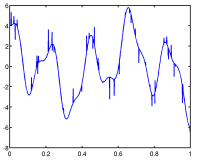Main»SPARCO: A toolbox for testing sparse reconstruction algorithms

Download:
v.1.2 (2 Jul 2008)
.zip (3.8MB)
.tgz (3.6MB)
tech report

## Introduction

Sparco is a suite of problems for testing and benchmarking algorithms for sparse signal reconstruction. It is also an environment for creating new test problems, and a suite of standard linear operators is provided from which new problems can be assembled. Sparco is implemented entirely in Matlab and is self contained. (A few optional test problems are based on the CurveLab toolbox, which can be installed separately.)

At the core of the sparse recovery problem is the linear system

Ax + r = b,

where A is an m-by-n linear operator and the m-vector b is the observed signal. The goal is to find a sparse n-vector x such that r is small in norm.

This technical report gives an overview of the Sparco toolbox.

## Quick start

The main interface to the test problems is through the `generateProblem` script found at the top-level directory. To instantiate a particular test problem, simply call `generateProblem` with the problem's ID number. For instance, problem five generates a signal from a few atoms in a DCT-Dirac dictionary:The signal generated by `generatePoblem(5)`
``` >> P = generateProblem(5);
```

This create a problem structure `P` which contains all the information needed to access this problem. The resulting structure contains many bits and pieces, including function handles and data vectors. For example,

``` P.A       a function handle to the operator A
P.b       the right-hand-side vector
P.sizeA   is a tuple with the number of rows and columns in A
```

The function handle P.A behaves as follows:

```   z = P.A( x, mode )  gives  z = A *x    if  mode == 1
z = A'*x    if  mode == 2
z = size(A) if  mode == 0.
```

It is also possible to use the `classOp` tool to instantiate the operator as an overloaded object, e.g.,

```>> C = classOp(P.A); b = P.b;
>> g = C'*b;  # Equivalent to g = P.A(b,2);
>> y = C *g;  # Equivalent to y = P.A(g,1);
```

The best way to get started is to browse the examples in the EXAMPLES subdirectory.

To get a full list of problem numbers, do

```>> plist = generateProblem('list');
```

## Related links

L1 solvers:

• FPC: Fixed-point continuation
• GPSR: Gradient projection for sparse reconstruction
• l1_ls: Simple Matlab Solver for l1-regularized Least Squares Problems
• SPGL1: A solver for large-scale sparse reconstruction

Related software:

Resources:

## Referencing Sparco

When referencing Sparco, please cite the following:

``` @techreport{sparco:2007,
Author = {E. {van den} Berg and M. P. Friedlander
and G. Hennenfent and F. Herrmann
and R. Saab and {\"O}. Y{\i}lmaz},
Institution = {Dept. Computer Science},
Address = {University of British Columbia, Vancouver},
Number = {TR-2007-20},
Title = {Sparco: {A} testing framework for sparse reconstruction},
Month = {October},
Year = {2007}
}
```

## Credits

Sparco is distributed under the GNU Public License.

We would be delighted to hear from you if you find Sparco useful, or if you have any suggestions, contributions, or bug reports. Please send these to

This research is supported in part by an NSERC Discovery Grant and by an NSERC Collaborative Research and Development Grant (334810-05) that funds the DNOISE project.